150810 Epaper

 A Section B SectionC Section D Section150809 Epaper

 A Section B SectionC Section D Section150808 Epaper

 A Section B SectionC Section D Section150807 Epaper

 A Section B SectionC Section D Section150806 Epaper

 A Section B SectionC Section D Section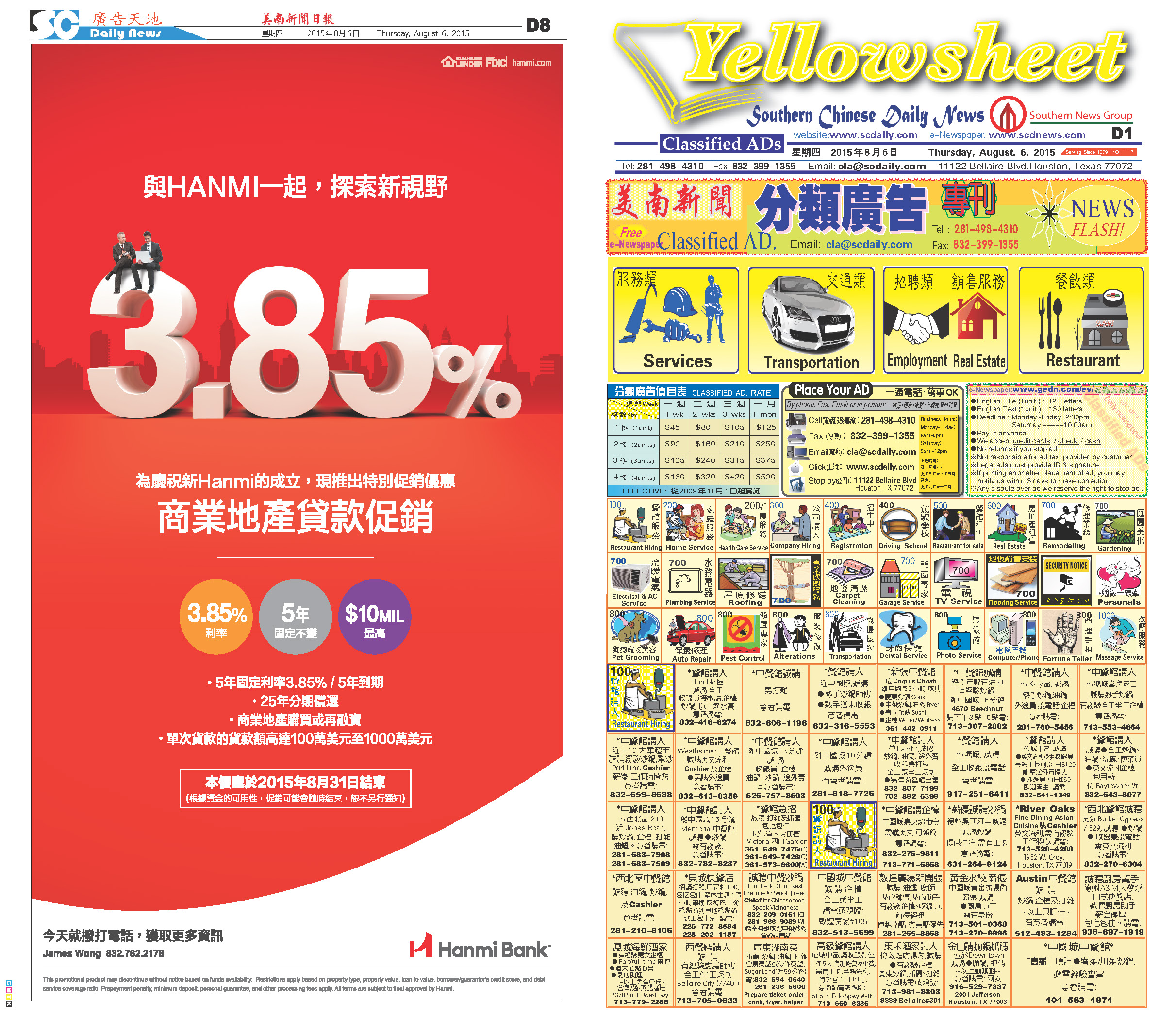150805 Epaper

 A Section B SectionC Section D Section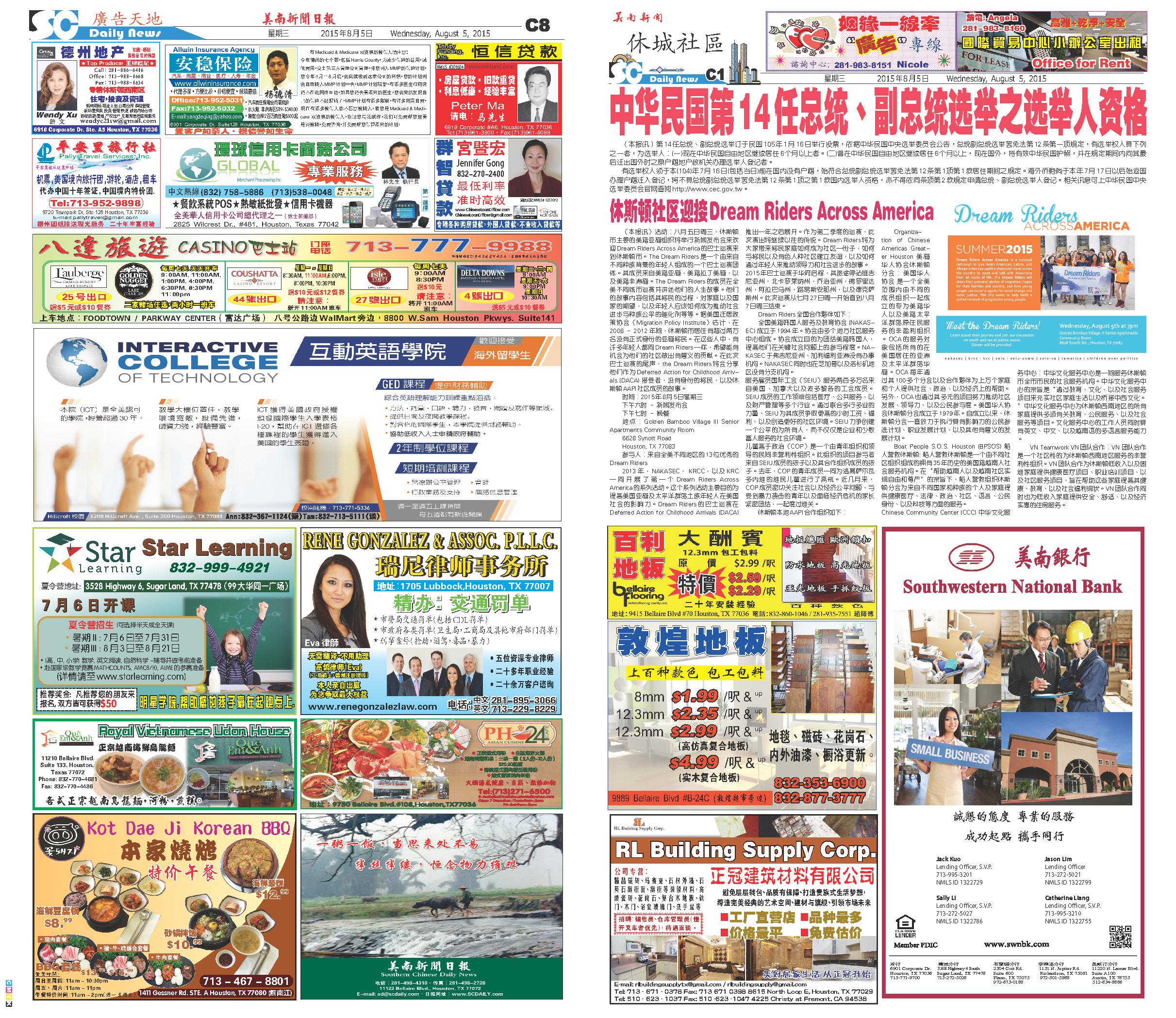150804 Epaper

 A Section B SectionC Section D Section150803 Epaper

 A Section B SectionC Section D Section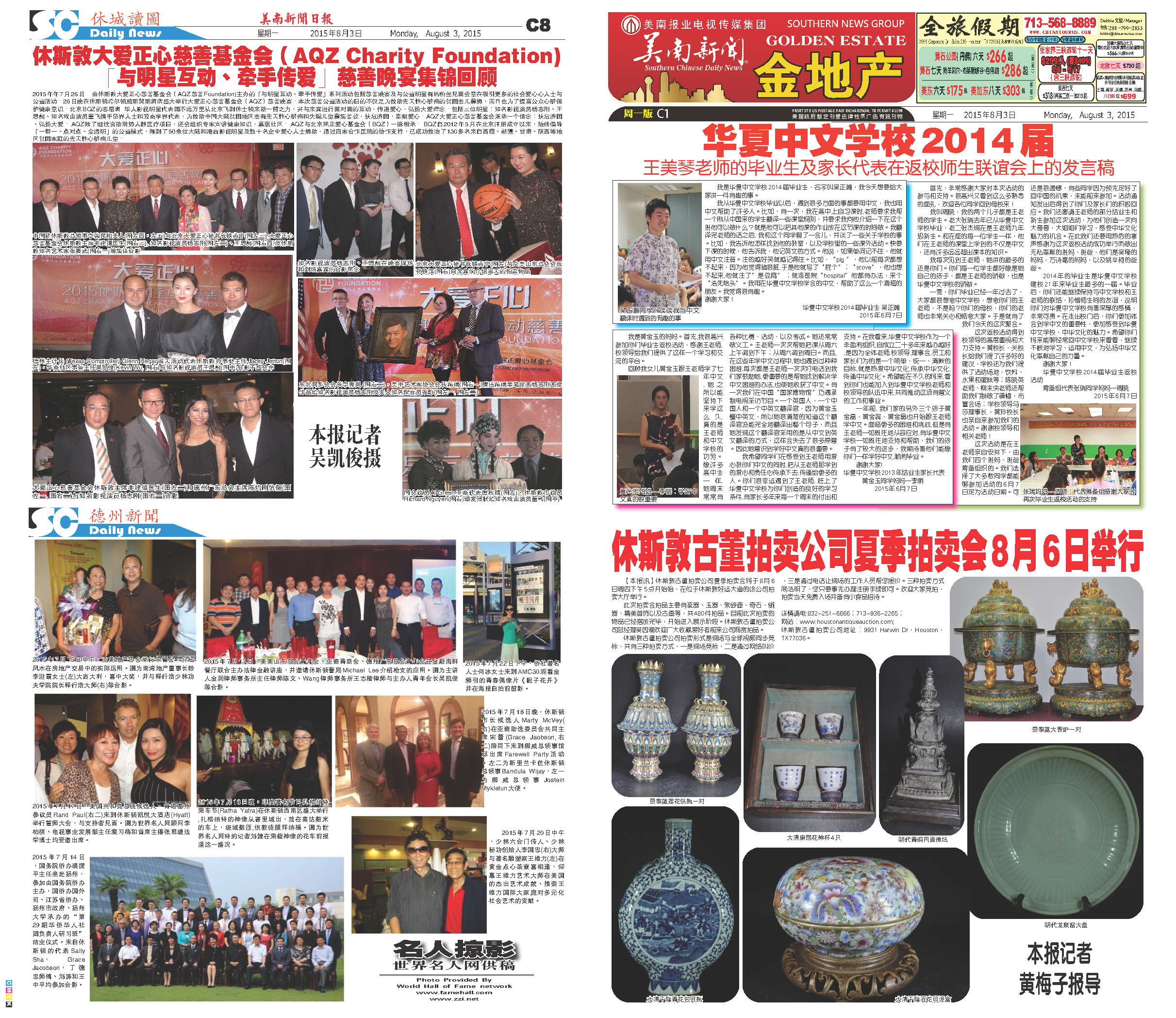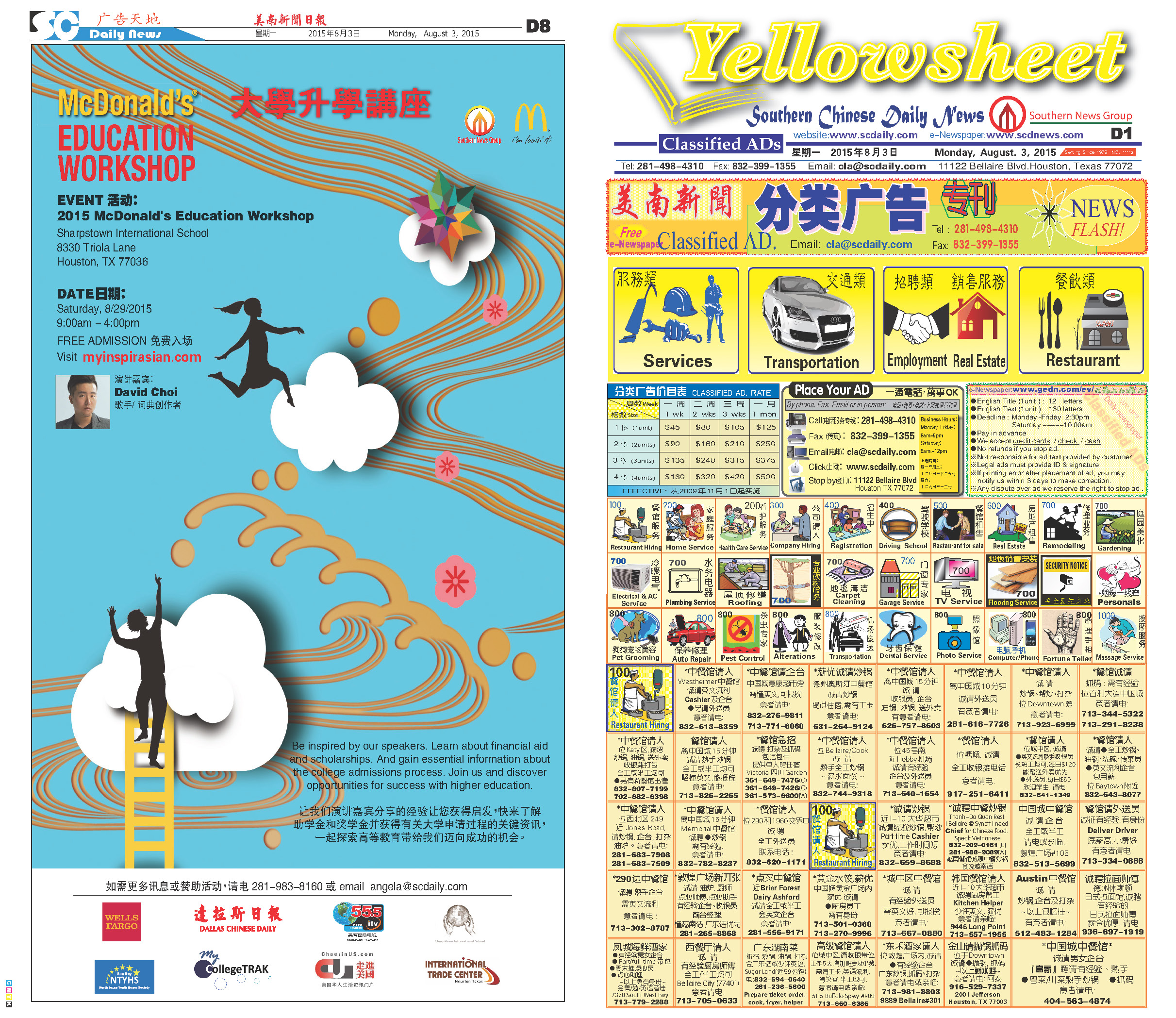150801 Epaper

 A Section B SectionC Section D Section150802 Epaper

 A Section B SectionC Section D Section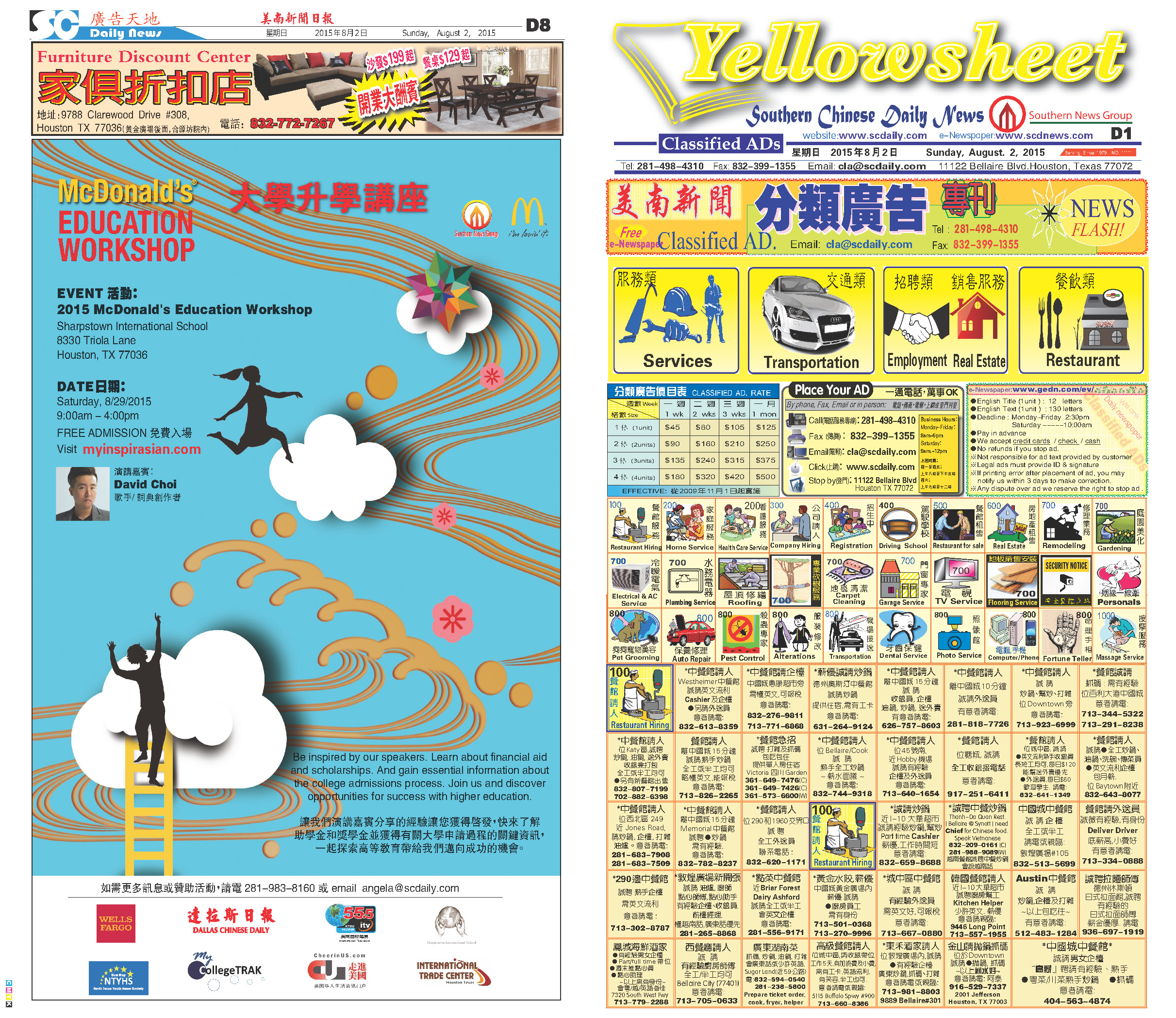150731 Epaper

 A Section B SectionC Section D Section